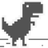# Holding Measurements To A Higher Standard

In Physics by Brian Koberlein3 Comments

When we make measurements of things in the universe, we can express the results in some type of units. The mass of a black hole in solar masses, or the distance to Jupiter in astronomical units, for example. But to be accurate, all of these units need to be based upon well measured standards that form the basis for all other units. Defining those standards poses certain challenges. After all, how to you measure something that is used to define your measurements?

Our modern foundation of measurements is the metric system (or more formally the International System of Units). In this system, seven base units are defined by convention (meter, kilogram, second, ampere, kelvin, mole and candela), and from these all other measuring units are derived. Even most non-metric units are formally defined by metric quantities. For example, an inch is defined as 2.54 centimeters. An English pint is exactly 568.26125 cubic centimeters.

The base units and their connection to physical constants. Credit: Wikipedia

Originally, the metric base units were defined in terms of physical quantities that could be measured. A kilogram was proposed as the mass of a liter (1000 cubic centimeters) of water. The meter was proposed by declaring that the distance from the north pole to the equator along a line through Paris is 10,000 kilometers. The second was defined in terms of a standard solar day being exactly 24 hours long. While these definitions initially worked well, as our measurements became more precise things became problematic. As measurement of the Earth’s circumference improved, the length of a meter would necessarily change. Since the volume of a liter is defined in terms of length, the mass of a kilogram likewise shifted. Precise measurements of Earth’s rotation showed that the length of a day varied, so even the second wasn’t entirely fixed.

A replica of the standard kilogram. Credit: Wikipedia

To solve this problem, some of the units were redefined in terms of a specific physical object. For example, in the 1870s the meter was redefined as the distance between two marks on the prototype meter bar made of platinum and iridium. In 1889 a cylinder of platinum-iridium alloy was defined as the prototype kilogram. While these were more precise than earlier definitions, they still had problems. As physical objects they need to be handled and measured in order to calibrate units. They can also change as they interact with their environment. We know, for example, that the standard kilogram varies in mass over time relative to official copies. These are small changes, on the order of micrograms, but they limit the precision of our measurements.

As we’ve studied the physical universe, we’ve found that the universe itself seems to have a set of absolute physical standards. Some things, such as the speed of light, the universal constant of gravity, and the charge of an electron, seem to have precise values that are absolute and unchanging throughout the universe. These physical constants could thus be used as the fundamental scale from which our metric system is defined. For some units this has already been done. In 1983 the speed of light was defined as exactly 299,792,458 m/s, and the second is defined in terms of the frequency of light from a caesium 133 atom. As a result, the meter is now defined in terms of the speed of light. But other units such as the kilogram are still based upon the 1889 prototype. There is currently a proposal to redefine the kilogram and other units in terms of physical constants. If the proposal passes, the metric system would no longer depend upon prototype objects.

But if we defined our units in terms of physical constants, what if those constants change over time? While this has been expressed as a concern, it isn’t really that much of a problem. We know from observations that the physical constants appear uniform across billions of years and billions of parsecs. All evidence so far shows them to be fixed and unchanging. And fixing our definitions in terms these physical values doesn’t prevent us from detecting the relative changes of these constants over time. So if we find they are changing we can always make new definitions for our units, just as we have in the past.

It would just be a matter of holding our measurements to a higher standard.

1.1.Author
2.